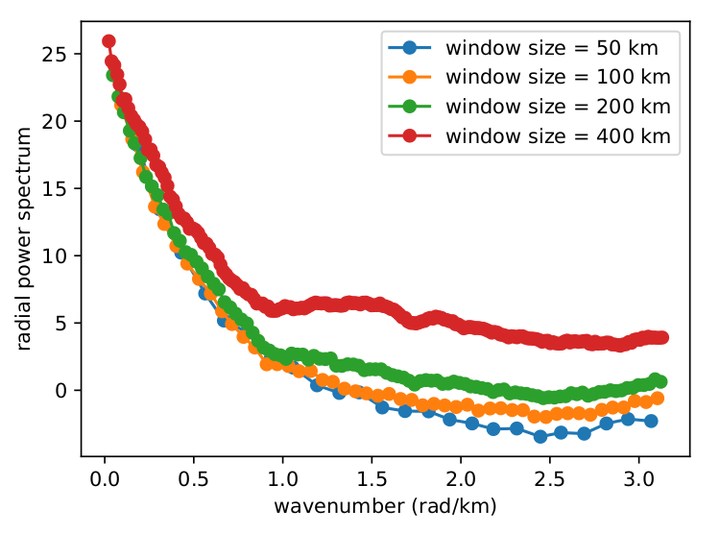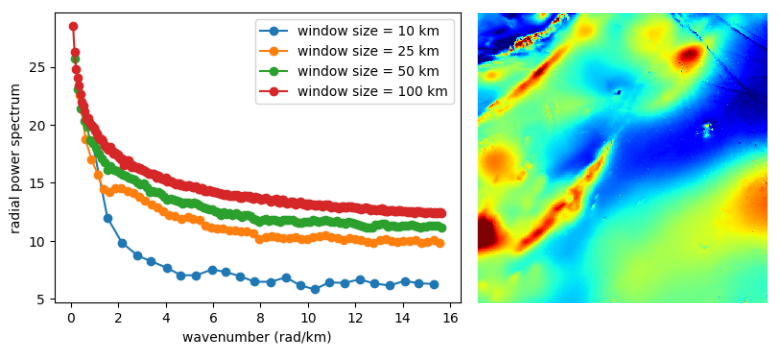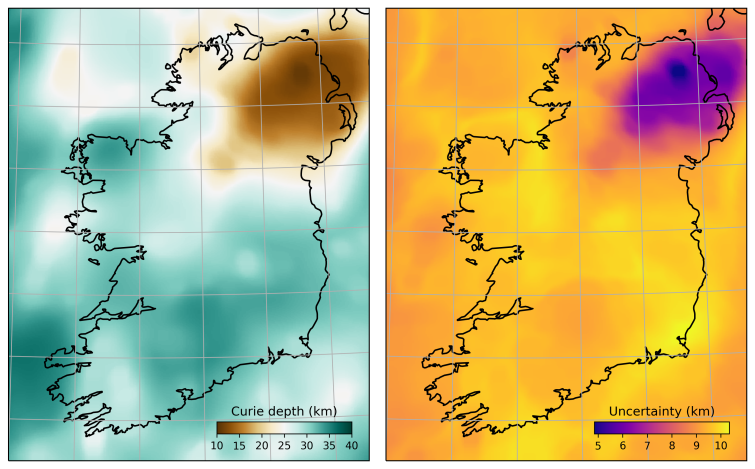# Curie point depthRadial power spectrum of the magnetic anomaly using different window sizes

Magnetic data is easy to come by on the surface of the Earth. It is relatively cheap to measure the magnetic anomaly using light aircrafts or drones, and susceptibility meters can be bundled along with gravity meters for additional prudence. Anomalies are assumed to reside on a horizontal surface at an elevation $z_t$ above magnetic sources.

As magnetite is the most magnetic mineral, the Curie depth is often interpreted to be the Curie point of magnetite - approximately 580C. Curie depth thus offers a very desirable isotherm in the lower crust.

Estimating the bottom of magnetic sources is usually accomplished by analysing the Fourier spectrum of magnetic anomalies. It’s computation is relatively simple:

## Step-by-step

1. Fast-Fourier transform (FFT) of the magnetic anomaly
2. Shift the zero-frequency component to the center of the spectrum
3. The gradient of the radial spectrum vs. wavenumbers of the power spectra obtains the Curie depthThe caveat here is that the radial power spectra will vary significantly on the choice of window size and fractal parameters. A synthetic power spectrum was derived by Bouligand et al. (2009), eq. 4. We minimise the residual between the computed power spectrum, $\phi_d$, for a given window using a FFT of the magnetic anomaly and our synthetic power spectrum, $\phi_m$, controlled by these unknown parameters:

• $\beta$ - a fractal parameter
• $z_t$ - the top of magnetic sources
• $\Delta z$ - the thickness of the magnetic layer

## Inverse problem

Gradient-based minimisation quickly converges to a model of minimum misfit between $\phi_d$ and $\phi_m$. Finite-difference updates to the Jacobian are feasible here since number of points in $\phi_d$ greatly outweights our four unknowns, thus the problem is well-posed; but this remains a unique solution in a very nonunique problem. $\beta$ exterts a much larger sensitivity to the shape of $\phi_m$ in the low frequency domain than $\Delta z$.

At this point our inverse problem is somewhat ugly: $\beta$ primarily controls the shape of the radial power spectrum, but we are only really interested in the value of $z_t$ and $\Delta z$. It transpires that $\beta$ is related to rock type, determined from borehole susceptibilities, and does not vary significantly throughout a study area. We can introduce a prior for $\beta$ to penalise its variation within an uncertainty range, thereby amplifying the sensitivity of the misfit function to $\Delta z$:

$$P(\mathbf{m}) = \frac{\parallel \beta (m) - \beta p \parallel^2}{\sigma_{\beta p}^2}$$

Curie depth computation involves two stages:

1. Ensure there is no a priori information and commence with suitable starting values for $\beta, z_t, \Delta z$.
2. Take the median and standard deviation of all parameters and add them as priors within the misfit function, then run the optimisation again.

## Sensitivity analysis

A sensitivity analysis is essential to gauge the amount of uncertainty in a Curie depth determination, given the highly non-unique nature of this inverse problem. We randomly perturb each of $\beta, z_t, \Delta z$ within their prior distributions and build an ensemble of Curie depth realisations. We can query the posterior for various quantities, such as the median and variance, and extract covariance information to quantify the variation of Curie depth with statistical rigour.Above is the Curie depth we calculated across Ireland using the EMAG2 global magnetic anomaly compilation. Download PyCurious and try the code out for yourself.##### Dr. Ben Mather
###### Computational Geophysicist

My research interests include Bayesian inversion, Python programming and Geodynamics.